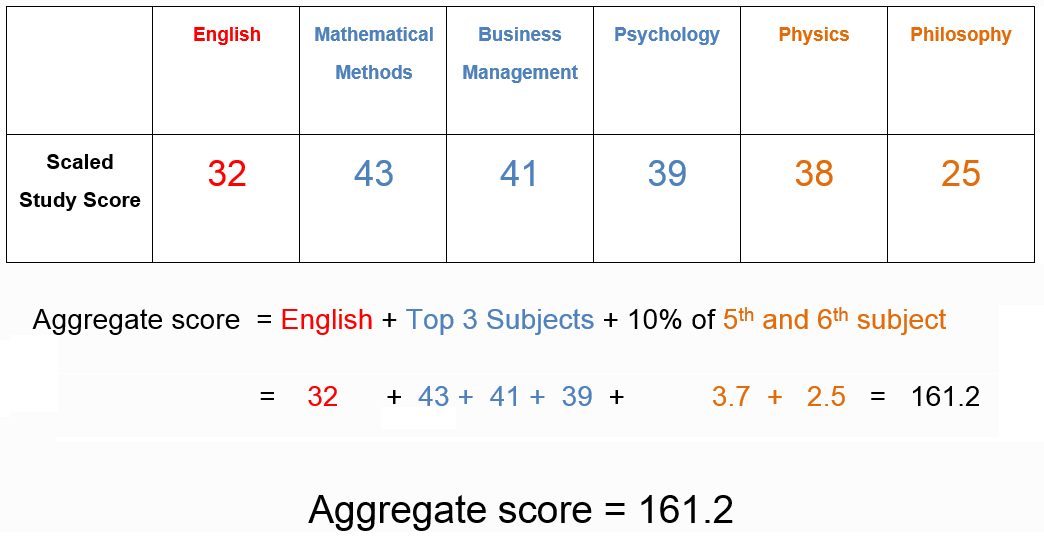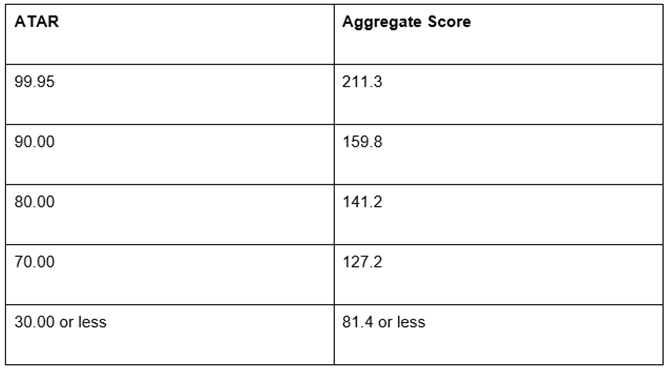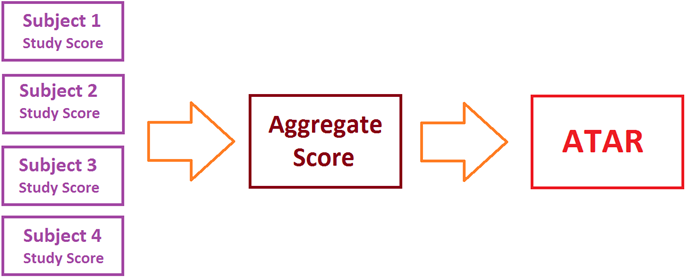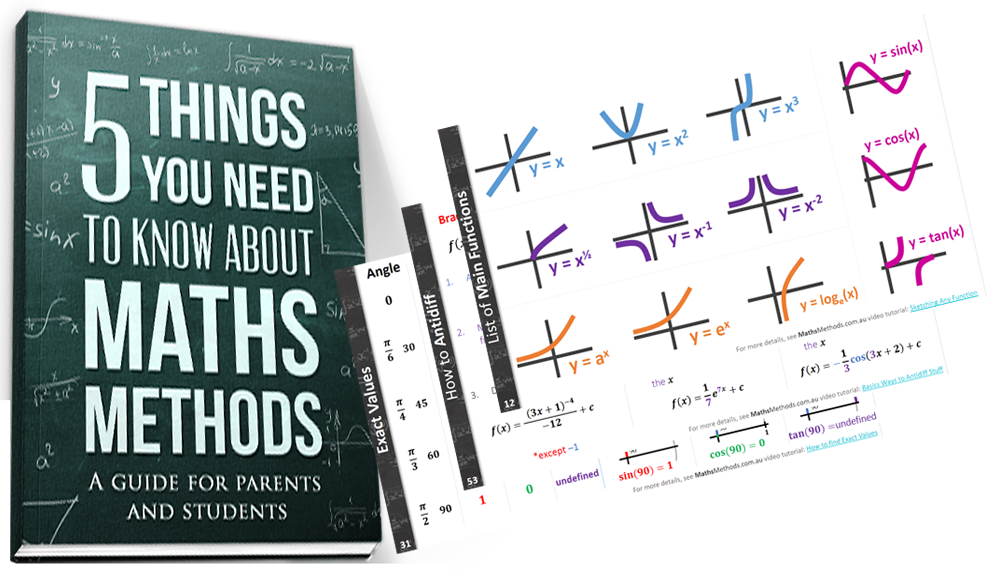# How is ATAR Calculated?

One of the big mysteries for students and parents is how the magical ATAR number is calculated. Here’s a brief overview of what is done.

## What is the ATAR?

The Australian Tertiary Admission Rank (ATAR) is a number between 30.00 and 99.95 with increments of 0.05 that represents a student’s rank within Victoria.

For example, if you score 99.95, this is akin to being in the top 0.05% of Victoria. Likewise, an ATAR score of 80.00 is indicative of being in the top 20%.

## Then what’s a Study Score?

The Study Score is value out of 50 which ranks a student’s performance for a particular VCE (Victorian Certificate of Education) subject. Completed year 12 subjects are each given a Study Score which are then used to calculate the ATAR. Let’s have a look at what certain study scores mean in terms of rank:

Standard Study Scores:

30 means in the top 50% of Methods students

35 is the top 26%

40 is top 9%

45 is the top 2%

## Scaled Study Score

Some subjects are more difficult than others to score well in. Scaling was introduced so that there is a level playing field and students can pick subjects they are interested in without worrying about how it will affect their ATAR. It changes slightly every year but let’s have a look at what a study score in Maths Methods is usually means:

Maths Methods Scaled Study Scores:

20 went to a 21

25 went to 28

30 went to 34

35 went to 40

40 went to 44

## Aggregate Score before ATAR

The word “aggregate” means something that is formed by several parts. For example, a cake is an aggregate because it is made from flour, milk, sugar etc.

The Aggregate score is calculated by adding the Scaled Study Scores of English, the three highest Scaled Study Scores and 10% of a fifth and sixth Study Score.## Finally, ATAR is calculated from the Aggregate Score

The ATAR score comes directly from the aggregate score. VTAC provides a table which shows the relationship between the two numbers. Here is an example from 2013.## The Overall Flow

This is the basic sequence used to calculate the ATAR score:## That’s the Basic Idea

This is the basic overview of how the ATAR Score is calculated. You may be interested in how SACs (School Assessed Coursework) contributes to ATAR, you can learn that by clicking here.

If you have any other questions, just send me an email at alex@mathsmethods.com.au.

Hope this helps 🙂

### Enjoyed this? Get more stuff emailed to your inbox!## Make Methods Less Stressful!